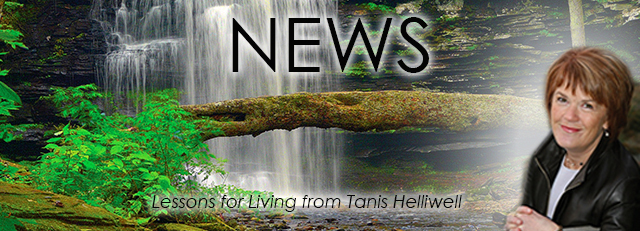# MARCH 2021

 /* styles */ Dear Friend,
 /* styles */ In honor of St. Patrick's Day this month, a new video from me to you on our relationships with elementals. And my leprechaun books are on sale this month, in English, French, Spanish and Dutch. We will be very busy working over the next weeks and months on some wonderful new ways of learning and co-creating together! One of these is my course Co-creating with Nature Spirits to Heal the Earth, which will soon be available as a self-study e-course for the first time.
 table div table+table+table+table+table div table{width:100%;padding:0}table div table+table+table+table+table div table img{width:96.23%;padding:0;float:none}table div table+table+table+table+table div table td{width:100%;padding:0 1.88% 18px}/* styles *//* styles */
 table div table+table+table+table+table+table+table div table{width:100%;padding:0}table div table+table+table+table+table+table+table div table img{width:96.23%;padding:0;float:none}table div table+table+table+table+table+table+table div table td{width:100%;padding:0 1.88% 18px}/* styles */## NEW VIDEO: Relationships with Elves, Leprechauns and other Elementals

 /* styles */ Many thanks to Li und Jan Temmel, who created this beautiful video. Alas, it may be a while before I can see you all again in person, but how wonderful to be able to share this experience with you now on video. The video is in English with simultaneous German translation. View it on Youtube here: https://youtu.be/EJbU67r3Zw4
 table div table+table+table+table+table+table+table+table+table+table div table td,table.module-9{width:100%;padding:0}table div table+table+table+table+table+table+table+table+table+table div table{width:100%;float:none;margin-left:auto;margin-right:auto;padding:0}table div table+table+table+table+table+table+table+table+table+table div table a{border:0 none;text-decoration:none}table div table+table+table+table+table+table+table+table+table+table div table img{width:100%!important;border:0 none;text-decoration:none}/* styles */
 table div table+table+table+table+table+table+table+table+table+table+table div table{width:100%;padding:0}table div table+table+table+table+table+table+table+table+table+table+table div table img{width:96.23%;padding:0;float:none}table div table+table+table+table+table+table+table+table+table+table+table div table td{width:100%;padding:0 1.88% 18px}/* styles */# 25% off paperbacks and e-books for March

My books Summer with the Leprechauns and Pilgrimage with the Leprechauns are required reading anytime, but especially now! IIT will be launching our Nature Spirits Self-Study e-Course soon (see the next newsletter item below this book sale), and these books may be very helpful for you in preparing you for the course. If you don't have a copy yet, or if you wish to share with friends and family, now is a good time to do so. My leprechaun series is available at a special price this month in English, French, Spanish and Dutch. (German editions are available at regular prices.)

# ENGLISH

Print & e-books 25% off

 table div table+table+table+table+table+table+table+table+table+table+table+table+table+table+table+table div table{width:100%;padding:0}table div table+table+table+table+table+table+table+table+table+table+table+table+table+table+table+table div table table{padding:0;float:left!important;width:52.075%!important}table div table+table+table+table+table+table+table+table+table+table+table+table+table+table+table+table div table table+table td,table div table+table+table+table+table+table+table+table+table+table+table+table+table+table+table+table div table td{padding-left:0;padding-right:0}table div table+table+table+table+table+table+table+table+table+table+table+table+table+table+table+table div table table td{padding-left:0;padding-right:20px}table div table+table+table+table+table+table+table+table+table+table+table+table+table+table+table+table div table table+table{float:left!important;width:47.925%!important}/* styles */
 table div table+table+table+table+table+table+table+table+table+table+table+table+table+table+table+table+table div table{width:100%;padding:0}table div table+table+table+table+table+table+table+table+table+table+table+table+table+table+table+table+table div table img{width:96.23%;padding:0;float:none}table div table+table+table+table+table+table+table+table+table+table+table+table+table+table+table+table+table div table td{width:100%;padding:0 1.88% 18px}/* styles */# SPANISH / DUTCH

25% off Spanish (print & e-book) & Dutch (e-book only)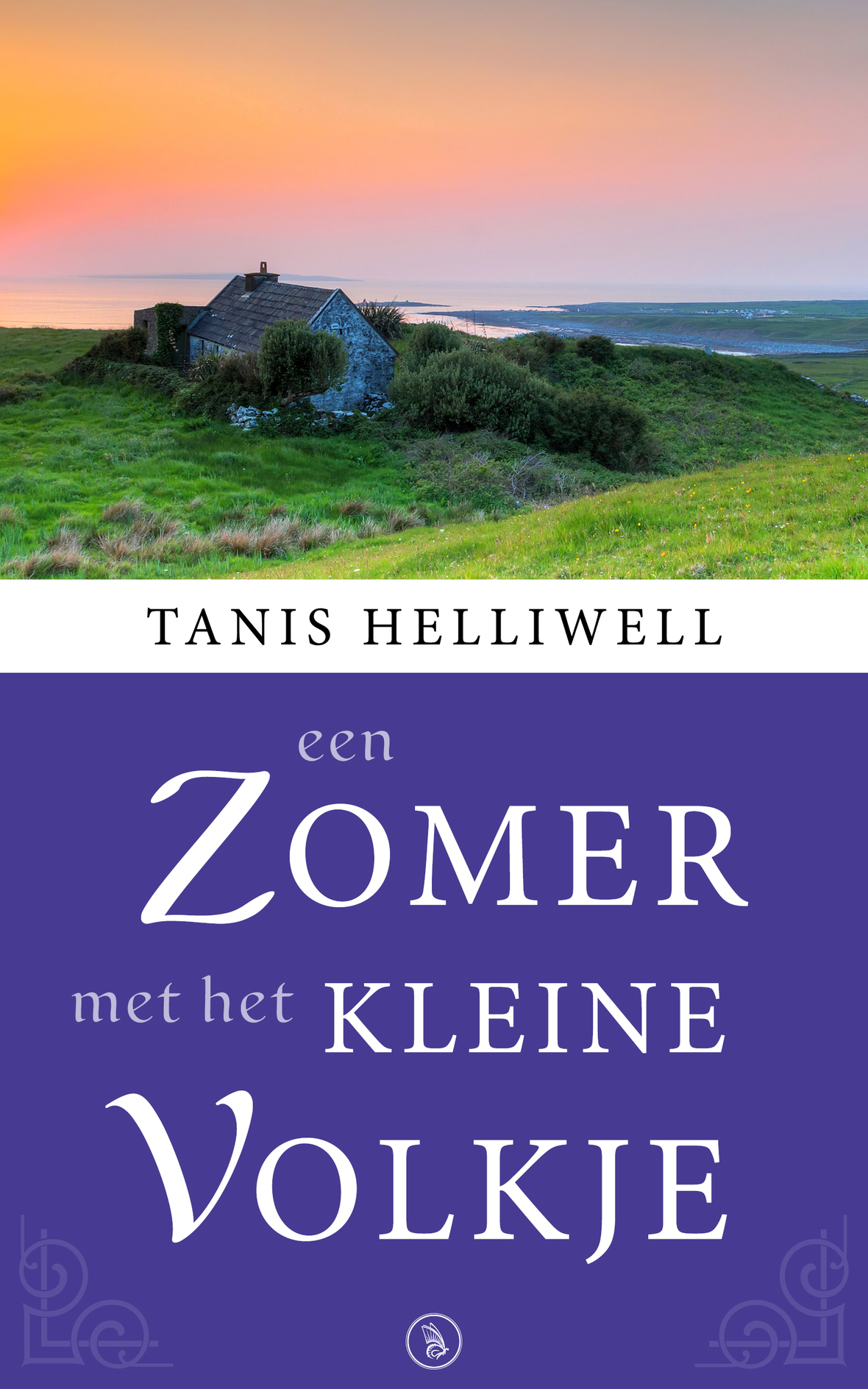table div table+table+table+table+table+table+table+table+table+table+table+table+table+table+table+table+table+table+table+table+table+table div table{width:100%;padding:0}table div table+table+table+table+table+table+table+table+table+table+table+table+table+table+table+table+table+table+table+table+table+table div table img{width:96.23%;padding:0;float:none}table div table+table+table+table+table+table+table+table+table+table+table+table+table+table+table+table+table+table+table+table+table+table div table td{width:100%;padding:0 1.88% 18px}/* styles */# FRENCH

25% off Un été avec les Leprechauns (e-book), & Pèlerinage avec les Leprechauns (print & e-book)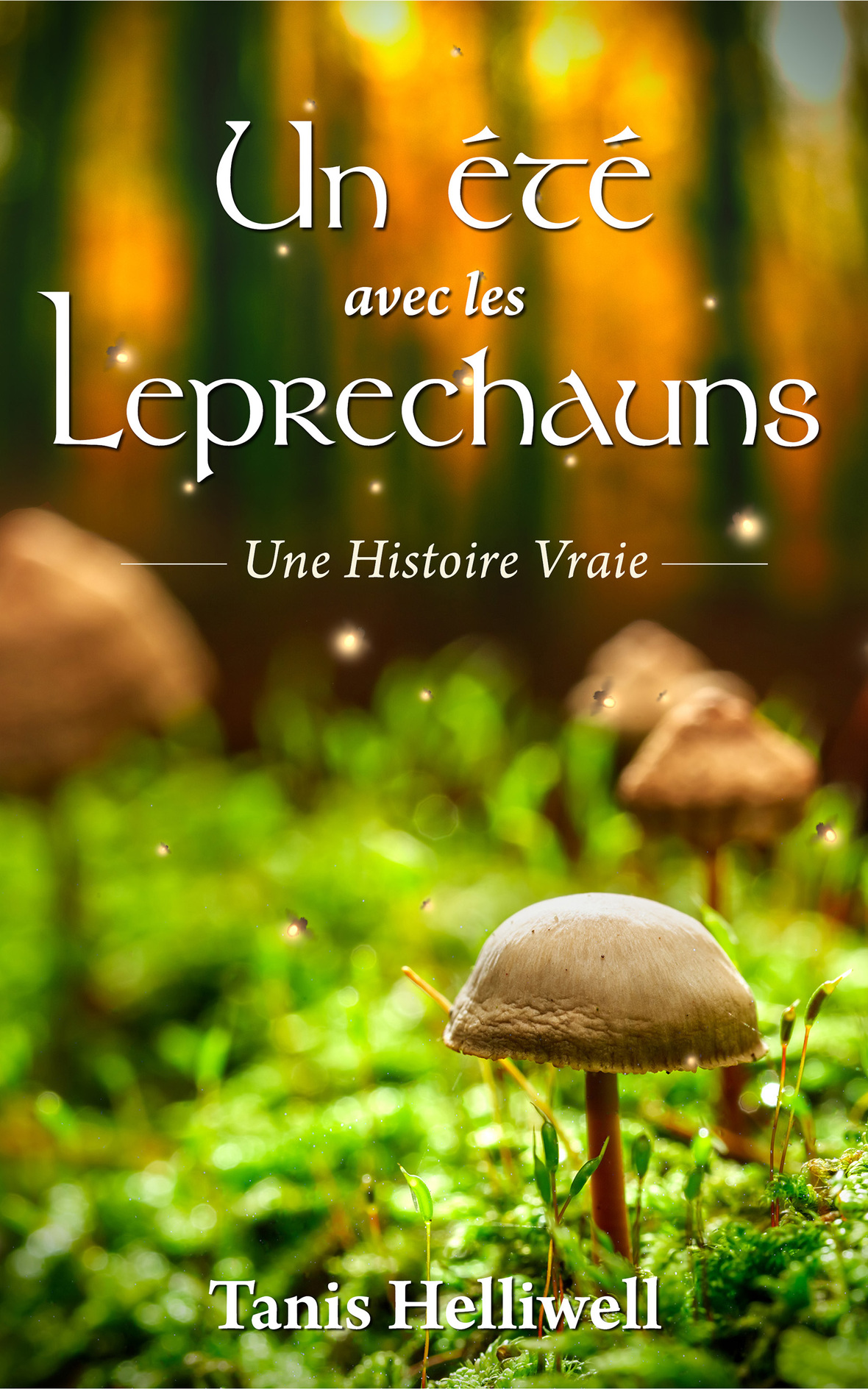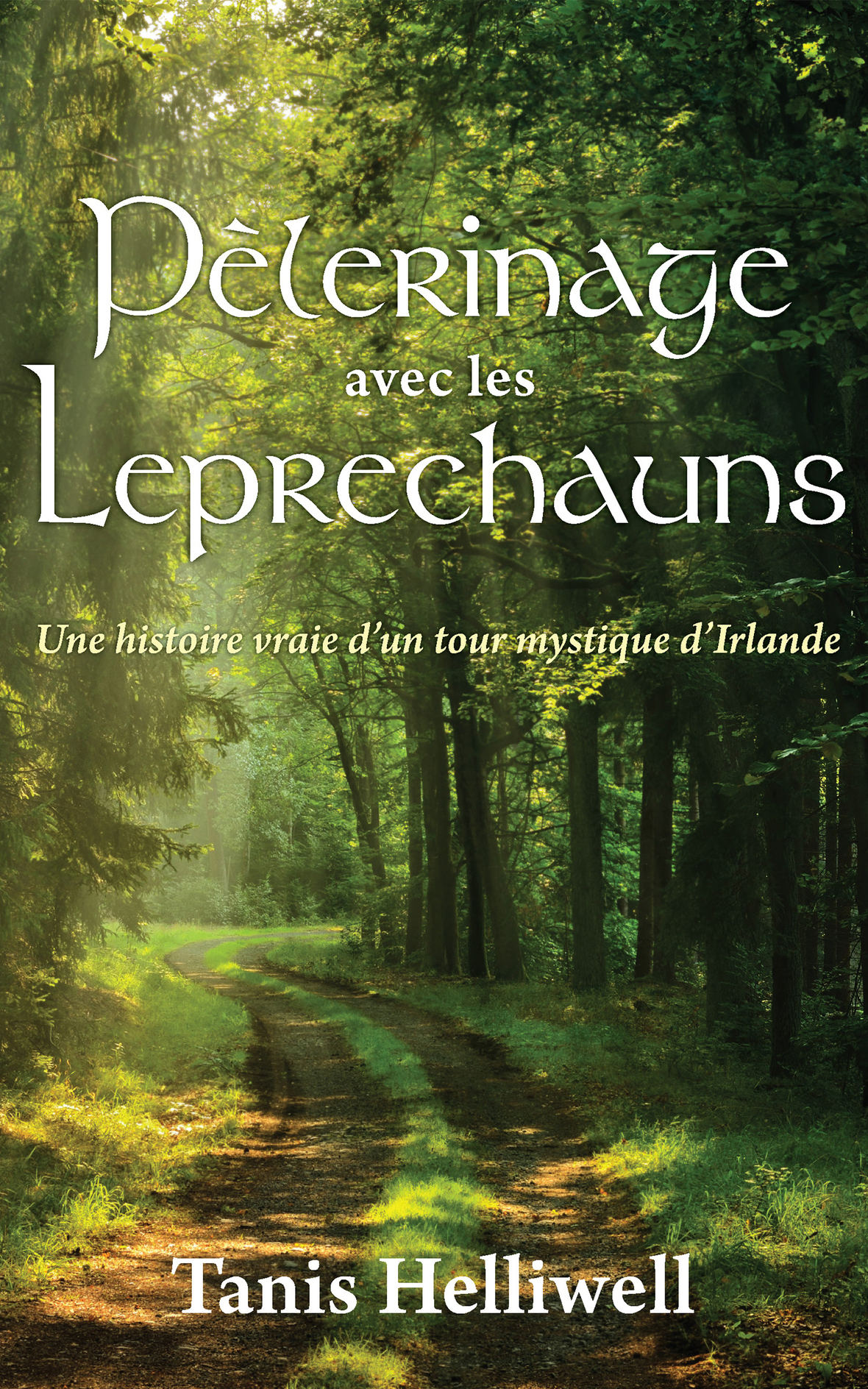table div table+table+table+table+table+table+table+table+table+table+table+table+table+table+table+table+table+table+table+table+table+table+table+table+table+table div table{width:100%;padding:0}table div table+table+table+table+table+table+table+table+table+table+table+table+table+table+table+table+table+table+table+table+table+table+table+table+table+table div table img{width:96.23%;padding:0;float:none}table div table+table+table+table+table+table+table+table+table+table+table+table+table+table+table+table+table+table+table+table+table+table+table+table+table+table div table td{width:100%;padding:0 1.88% 18px}/* styles */## NEW E-COURSE LAUNCHING SOON

 /* styles */ This new self-study e-course edition will assist folks from around the world who are interested in co-creating with nature spirits to restore themselves and the world to health.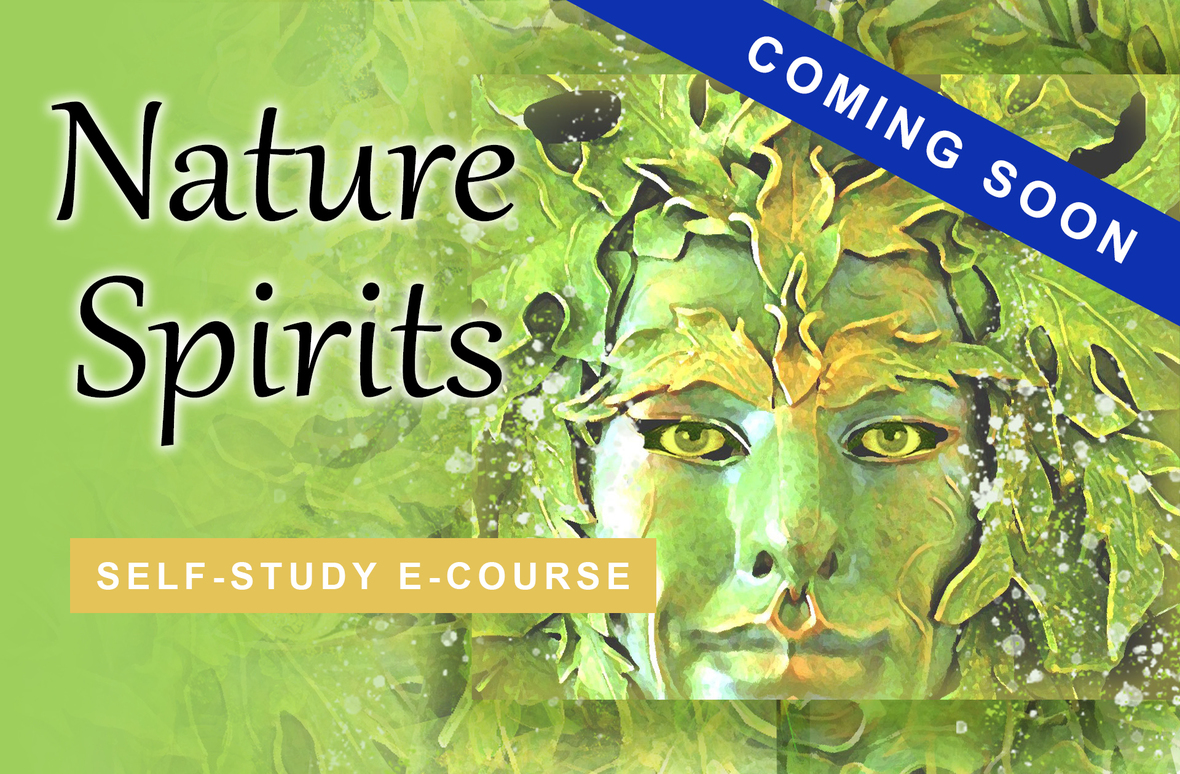▪ Learn about leprechauns, fairies, elves, and other nature spirits and how to connect with them.
▪ Meet one that wants to work with you.
▪ Learn how to create sanctuaries for elementals in your garden, apartment, natural space and in your heart.
▪ Discover how to balance the elements of earth, air, fire and water in your physical body for health and energy.
▪ Meet your Body Elemental, also called your Body Spirit, and learn how to heal and transform yourself with its assistance.
▪ Create a beautiful healthy Earth in co-operation with nature spirits.
▪ Learn how to flow through this current transitional time with ease and grace to manifest your full potential.
 ▪ Learn about leprechauns, fairies, elves, and other nature spirits and how to connect with them.
 ▪ Meet one that wants to work with you.
 ▪ Learn how to create sanctuaries for elementals in your garden, apartment, natural space and in your heart.
 ▪ Discover how to balance the elements of earth, air, fire and water in your physical body for health and energy.
 ▪ Meet your Body Elemental, also called your Body Spirit, and learn how to heal and transform yourself with its assistance.
 ▪ Create a beautiful healthy Earth in co-operation with nature spirits.
 ▪ Learn how to flow through this current transitional time with ease and grace to manifest your full potential.

The information covered in this new e-course has only been available previously at Tanis Helliwell’s in-person workshops or her live online course. This new self-study program will include four extensive workbook modules (plus a bonus module on "Fulfill Your Destiny as a Guardian of the Earth"!), plus MP3 audio downloads and video recordings.

Stay tuned for updates!

 table div table+table+table+table+table+table+table+table+table+table+table+table+table+table+table+table+table+table+table+table+table+table+table+table+table+table+table+table+table+table+table div table{width:100%;padding:0}table div table+table+table+table+table+table+table+table+table+table+table+table+table+table+table+table+table+table+table+table+table+table+table+table+table+table+table+table+table+table+table div table img{width:96.23%;padding:0;float:none}table div table+table+table+table+table+table+table+table+table+table+table+table+table+table+table+table+table+table+table+table+table+table+table+table+table+table+table+table+table+table+table div table td{width:100%;padding:0 1.88% 18px}/* styles */## Send in your photos

 /* styles */ A very happy St. Patrick's Day to you and your loved ones for 2021. "May you be touched by a bit of Irish luck today!" Do you have an image of a leprechaun, gnome or another elemental? We would love to add it to the Leprechaun Website. Send it in to info(at)iitransform(dot)com, or post it on Instagram using the hashtag #tanisleprechaun (remember to make it public) and we'll share it in our next IIT newsletter, too. I'm looking forward to seeing your photos.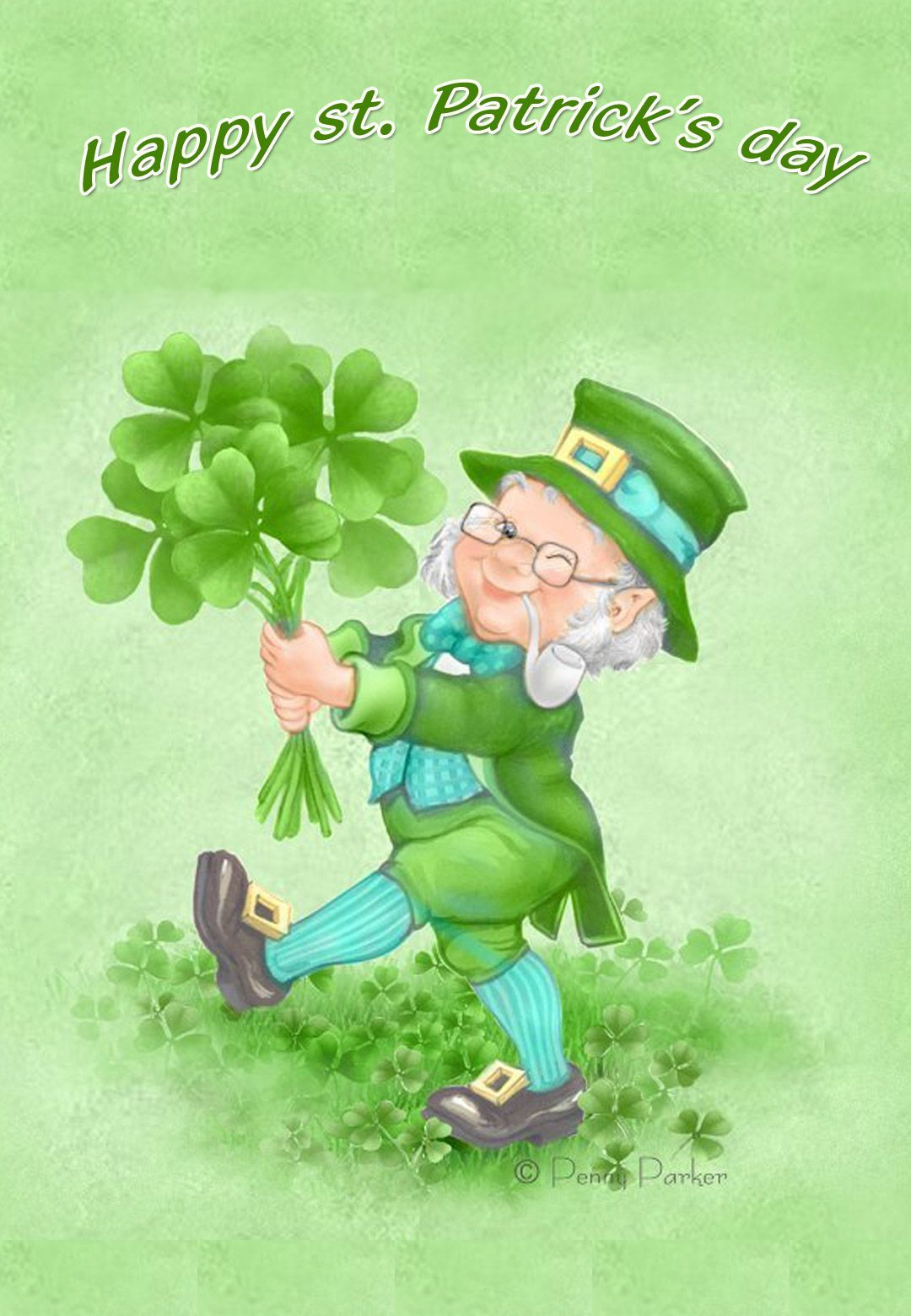table div table+table+table+table+table+table+table+table+table+table+table+table+table+table+table+table+table+table+table+table+table+table+table+table+table+table+table+table+table+table+table+table+table+table+table div table{width:100%;padding:0}table div table+table+table+table+table+table+table+table+table+table+table+table+table+table+table+table+table+table+table+table+table+table+table+table+table+table+table+table+table+table+table+table+table+table+table div table img{width:96.23%;padding:0;float:none}table div table+table+table+table+table+table+table+table+table+table+table+table+table+table+table+table+table+table+table+table+table+table+table+table+table+table+table+table+table+table+table+table+table+table+table div table td{width:100%;padding:0 1.88% 18px}/* styles *//* styles */ Love as always,/* styles */ Tanis Helliwell info@iitransform.com International Institute for Transformation
 Like   Tweet   Pin   in